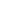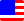Documents  >  Tutorial  >  Priority 3
Priority 3 operators

It describes the following operators with priority 3.

 + +x Unary + operator - -x Unary - operator ! !x Logical negation operator ++ ++x Prefix ++ operator -- --x Prefix -- operator

They are unary prefix operators.

Unary + operator

Unary - operator

Logical negation operator<

Prefix ++ operator

Prefix -- operator

Unary + operator

The unary + operator returns a new instance with the same value and same sign as the right operand of the operator.

The operand must be the int, long, or real class. Otherwise, an exception will be thrown.

int i = 10;

int j = i; // i and j refer to same instance.

int k = +i; // +i returns a new instance. Therefore, i and k refer to different instances.

If the operand returns the proxy class, the entity of the proxy is automatically used. The result is the same as calling the Entity getter of the proxy class.

int i = 10;

proxy pro = new proxy(i);

int j = +pro; // j is 10.

Unary - operator

The unary - operator returns a new instance with the same value and opposite sign as the right operand of the operator.

The operand must be the int, long, or real class. Otherwise, an exception will be thrown.

int i = 10;

int j = -i; // j is -10.

If the operand returns the proxy class, the entity of the proxy is automatically used. The result is the same as calling the Entity getter of the proxy class.

int i = 10;

proxy pro = new proxy(i);

int j = -pro; // j is -10.

Logical negation operator

The logical negation operator returns a new instance that inverted the boolean value of the right operand of the operator.

The operand must be the bool class. Otherwise, an exception will be thrown.

bool b = true;

bool c = !b; // c is false.

If the operand returns the proxy class, the entity of the proxy is automatically used. The result is the same as calling the Entity getter of the proxy class.

bool b = true;

proxy pro = new proxy(b);

bool b2 = !pro; // b2 is false.

Prefix ++ operator

The prefix ++ operator increments the value of the operand by 1 and then returns a new instance with the same value as the operand.

The operand must be one of the int, long, or real classes. Using the prefix ++ operator on any other class will throw an exception.

Even if the operand is the maximum value for that class, the prefix ++ operator will not cause an overflow. It will be the minimum value of the class.

int i = 10;

int j = ++i; // j is 11. i is 11.

If the operand returns the proxy class, the entity of the proxy is automatically used. The result is the same as calling the Entity getter of the proxy class.

int i = 10;

proxy pro = new proxy(i);

int j = 10 + ++pro; // j is 21. i is decremented.

Prefix -- operator

The prefix -- operator decrements the value of the operand by 1 and then returns a new instance with the same value as the operand.

The operand must be one of the int, long, or real classes. Using the prefix -- operator on any other class will throw an exception.

Even if the operand is the minimum value for that class, the prefix -- operator will not cause an overflow. It will be the maximum value of the class.

int i = 10;

int j = --i; // j is 9. i is 9.

If the operand returns the proxy class, the entity of the proxy is automatically used. The result is the same as calling the Entity getter of the proxy class.

int i = 10;

proxy pro = new proxy(i);

int j = 10 + --pro; // j is 19. i is decremented.